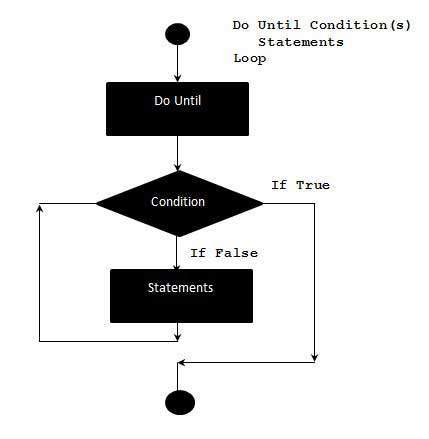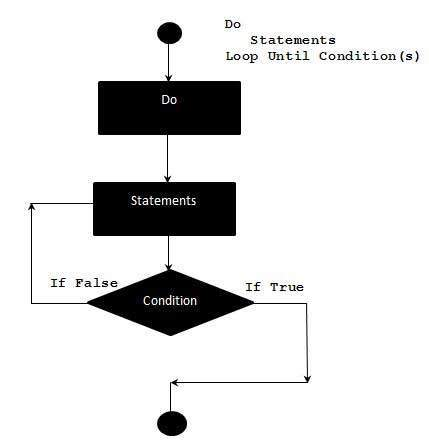# Do..Until Loops in VBScript

A Do..Until loop is used when we want to repeat a set of statements as long as the condition is false. The Condition may be checked at the beginning of the loop or at the end of loop.

## Syntax

The syntax of a Do..Until loop in VBScript is −

```Do Until condition
[statement 1]
[statement 2]
...
[statement n]
[Exit Do]
[statement 1]
[statement 2]
...
[statement n]
Loop
```

## Flow Diagram## Example

The below example uses Do..Until loop to check the condition at the beginning of the loop. The Statements inside the loop are executed only if the condition is false. It exits out of the loop when the condition becomes true.

```<!DOCTYPE html>
<html>
<body>
<script language = "vbscript" type = "text/vbscript">
i = 10
Do Until i>15  'Condition is False.Hence loop will be executed
i = i + 1
Document.write("The value of i is : " & i)
Document.write("<br></br>")
Loop

</script>
</body>
</html>
```

When the above code is executed, it prints the following output in the console.

```The value of i is : 11

The value of i is : 12

The value of i is : 13

The value of i is : 14

The value of i is : 15

The value of i is : 16
```

## Alternate Syntax

There is also an alternate Syntax for Do..Until loop which checks the condition at the end of the loop. The Major difference between these two syntax is explained below with an example.

```Do
[statement 1]
[statement 2]
...
[statement n]
[Exit Do]
[statement 1]
[statement 2]
...
[statement n]
Loop Until condition
```

## Flow Diagram## Example

The below example uses Do..Until loop to check the condition at the end of the loop. The Statements inside the loop are executed at least once even if the condition is True.

```<!DOCTYPE html>
<html>
<body>
<script language = "vbscript" type = "text/vbscript">
i = 10
Do
i = i + 1
Document.write("The value of i is : " & i)
Document.write("<br></br>")
Loop Until i<15 'Condition is True.Hence loop is executed once.

</script>
</body>
</html>
```

When the above code is executed, it prints the following output in the console.

```The value of i is : 11
```
vbscript_loops.htm